#### Vol. 33, No. 1, 1970

 Download this articleFor screen For printingRecent Issues Vol. 325: 1 Vol. 324: 1  2 Vol. 323: 1  2 Vol. 322: 1  2 Vol. 321: 1  2 Vol. 320: 1  2 Vol. 319: 1  2 Vol. 318: 1  2Online Archive Volume: Issue:The Journal Subscriptions Editorial Board Officers Contacts Submission Guidelines Submission Form Policies for Authors ISSN: 1945-5844 (e-only) ISSN: 0030-8730 (print) Special Issues Author Index To Appear Other MSP Journals
On C, 1 summability factors of Fourier series at a given point

### Fu Cheng Hsiang

Vol. 33 (1970), No. 1, 139–147
##### Abstract

Let f(αj) be a function integrable in the sense of Lebesgue over the interval (π,π) and periodic with period 2π. Let its Fourier series be

 f(x) ∼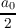+ ∑ n=1∞(a n cosnx + bn sinnx) ≡∑ n=0∞A n(x).
Whittaker proved that the series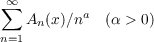is summable |A| almost everywhere. Prasad improved this result by showing that the series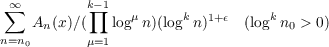is summable |A| almost everywhere.

In this note, the author is interested particularly in the |C,1| summability factors of the Fourier series at a given point x0.

Write

 φ(t) = f(x0 + t) + f(x0 − t) − 2f(x0),Φ(t) = ∫ 0t|φ(u)|du.
The author establishes the following theorems.

Theorem 1. If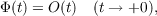then the series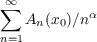is summable |C,1| for every α > 0.

Theorem 2. If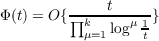as t +0, then the series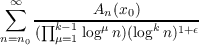is summable |C,1| for every 𝜖 > 0.

Primary: 42.20
##### Milestones